# Team:Duke/Modeling/Thermodynamic Model Application

•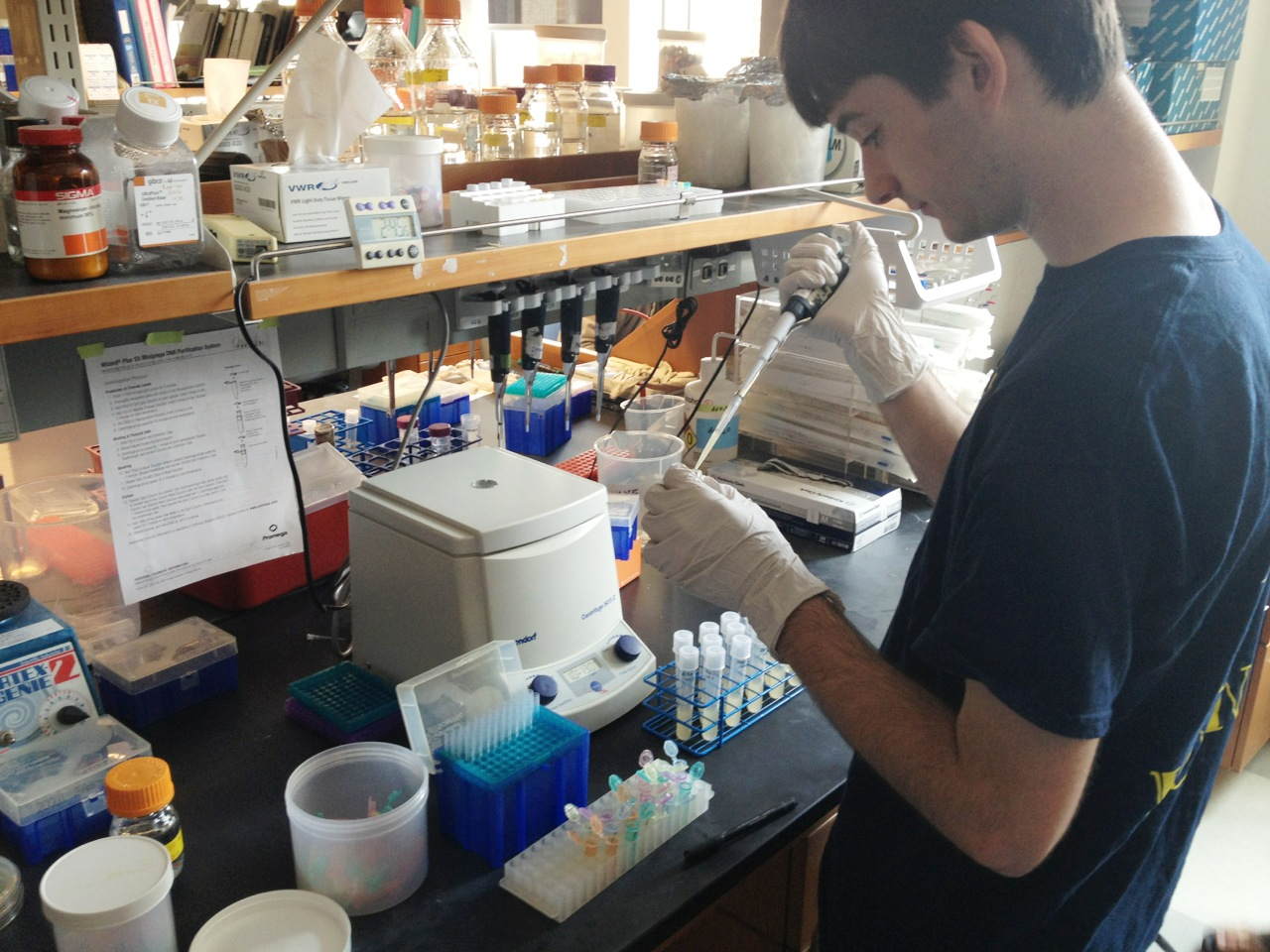•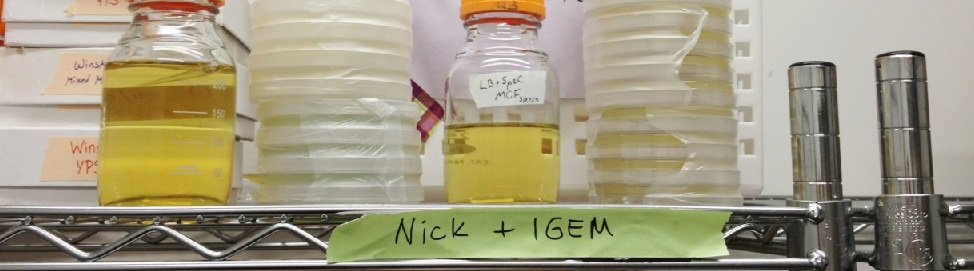•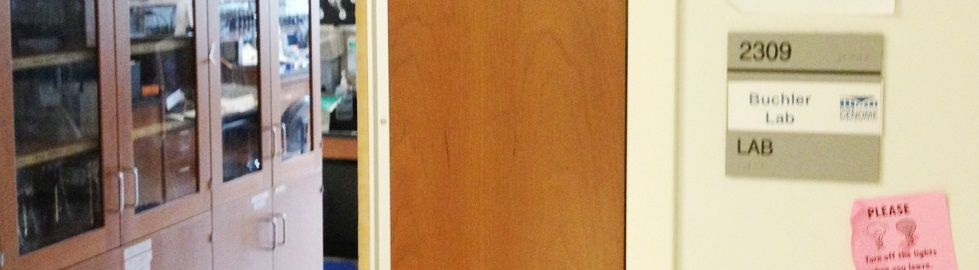•••••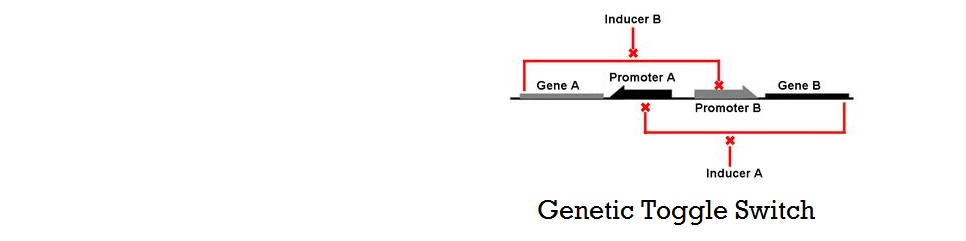•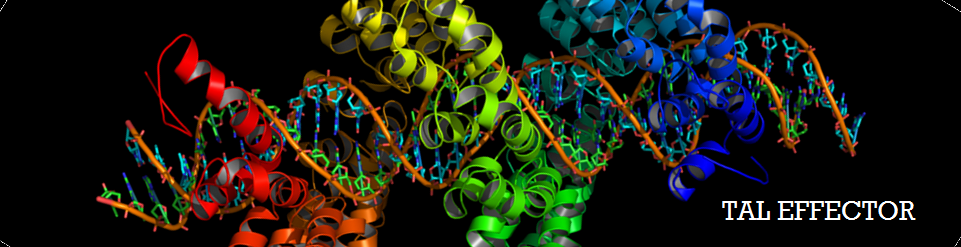•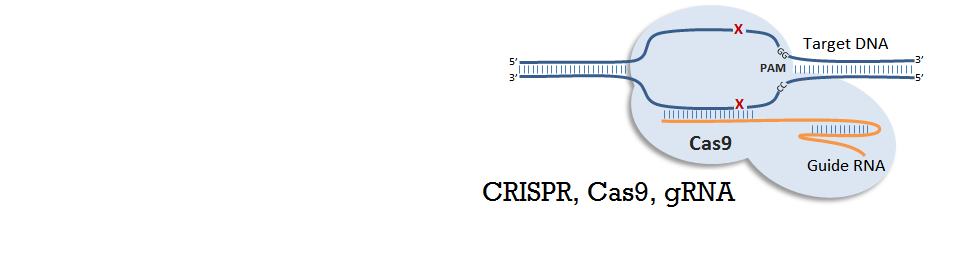# Thermodynamic Model of Cooperative Repression

To recap the derivation from the previous section, the key equation for thermodynamic model of cooperative repression is:

Recap of Thermodynamic Model

In this section, the above model will be explored; specifically, the relationship between the red-colored terms will be studied. First, the relationship between Pbound and the number of Freg's will show the effect of changing number of binding sites on level of gene expression. Then, the relationship between Pbound and KSrd (Dissociation constant of repressor bound to DNA) will show the effect of changing binding strengths of repressors on level of gene expression.

### Gene Expression Level vs. Number of Repressor Binding Sites

Figure 1. Relationship Between Pbound vs. Number of Freg's

As highlighted in the equation shown above, the relationship between Pbound and the number of Freg's was studied. The variables and their values used are summarized below.

Table 3.3.1. Meaning and Value of Variables Used

First, the basal level of Pbound was calculated using Freg = 1 (no Transcription Factors). Then, the Pbound values for the three cases--1 binding site, 3 binding sites, and 5 binding sites--were calculated for repressor level from 0.1 to 10,000 molecules/cell. Then finally, the Pbound values at these three cases were divided by the basal Pbound value to find the fold change in Pbound for the three cases. The results from these steps are graphed below.

Figure 3.3.2. Graph of Fold Change in Pbound vs. Number of Binding Sites

The black, blue, and red data points indicate the fold change in Pbound when 1, 3 and 5 repressor binding sites are present. Qualitatively, it clearly looks like the slope of the hill function becomes steeper with additional binding sites. When a hill function was fitted onto these data points using "cftool" command on MATLAB, it was quantitatively shown that the apparent Hill coefficient increases from 1.00 to 2.99 then to 4.86 as the number of binding sites increased from 1 to 3, then to 5.

The significance of the result above is that a non-Hill equation that models the effect of multiple repressor sites on the probability of RNAP binding demonstrated increase in cooperativity with increasing number of binding sites.

### Gene Expression Level vs. Binding Strength of Repressors

Figure 3.3.3. Relationship Between Pbound vs. Kd

Similarly, the relationship between Pbound and the dissociation constants of repressors was studied in this section. In addition to the variables and the values from previous section, a new set of variables were introduced to distinguish between weak and strong repressors. A value of 0.1nM was used for "Kd,strong" and this was the dissociation constant for strong repressors. Also, 10nM was used for "Kd,weak," which wass the dissociation constant for weak repressors. Because the strength of binding is represented as the "dissociation" constant, higher value translates to equilibrium shifting towards dissociated repressor ([DNA]+[Repressor]) instead of bound repressor ([DNA-Repressor]).

Just like in the previous section, the basal level of Pbound was calculated using Freg = 1 (no Transcription Factors). The key difference was that there were two types of Fregs: one for strong binding and one for weak binding. The effect of different combinations of weak and strong binding was demonstrated by plotting the fold change in Pbound for 5 Binding sites for the following cases:

1. 5 Strong Binding, 0 Weak Binding : Freg, total = (Freg, strong)5
2. 4 Strong Binding, 1 Weak Binding : Freg, total = (Freg, strong)4 * (Freg, weak)
3. 3 Strong Binding, 2 Weak Binding : Freg, total = (Freg, strong)3 * (Freg, weak)2
4. 2 Strong Binding, 3 Weak Binding : Freg, total = (Freg, strong)2 * (Freg, weak)3
5. 1 Strong Binding, 4 Weak Binding : Freg, total = (Freg, strong) * (Freg, weak)4
6. 0 Strong Binding, 5 Weak Binding : Freg, total = (Freg, weak)5

The result of having different combinations of strong and weak binding for a promoter with 5 binding site is plotted below. Plots on the left have more strong binding sites (Black = 5 Strong, 0 Weak), and plots on the right have more weak binding sites (Pink = 0 Strong, 5 Weak).

Figure 3.3.4. Graph of Fold Change in Pbound vs. Combination of Weak (10nM) and Strong (0.1nM) Binding

Again, when a Hill function was fitted onto these data points using "cftool" command on MATLAB, the apparent Hill coefficient were determined and labeled next to each plot. As shown, the apparent Hill coefficient is lowest when the degree of unbalanced binding strengths is greatest, and is higher at both ends where the binding strengths at the five binding sites are equal.

An important conclusion that can be drawn from this result is that balanced repressor binding strengths among the battery of repressor binding sites is crucial for increasing cooperativity. Also, even though the dissociation constant of weak repressors was hundred times higher than that of strong repressors, the difference in apparent Hill coefficients was relatively small.

Now that we have demonstrated the effect of number of binding sites and binding strengths on cooperativity using a thermodynamic model, we now move on to characterizing the effect of several parameters on the stability of a system using a Kinetic Model.

### MATLAB Code

The MATLAB codes used for the thermodynamic model can be found here.